×#### Thank you for registering.

One of our academic counsellors will contact you within 1 working day.

Click to Chat

1800-1023-196

+91-120-4616500

CART 0

• 0

MY CART (5)

Use Coupon: CART20 and get 20% off on all online Study Material

ITEM
DETAILS
MRP
DISCOUNT
FINAL PRICE
Total Price: Rs.

There are no items in this cart.
Continue Shopping```Important Results in Context with Rotation(i) arg z = 0 represents points (non-zero) on a ray emanating from origin making an angle θ with positive direction of real axis.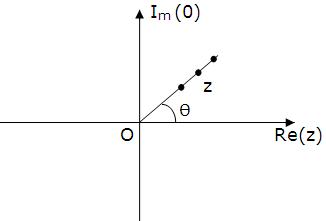(ii) arg (z-z1)= 0 represents points ( ≠3,) on ray emanating from z, making an angle θ with positive direction of real axis.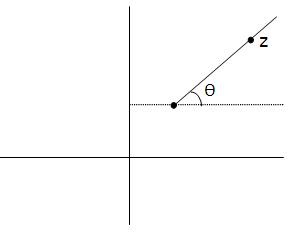Rotation theorem:

(i) If P(z1) and Q(z2) are two complex numbers such that |z1| = |z2|, then z2=z1 eiθ,  where θ = ⁄POQ.

(ii) If P(z1), Q(z2) and R(z3) are three complex numbers and ⁄PQR=Q, then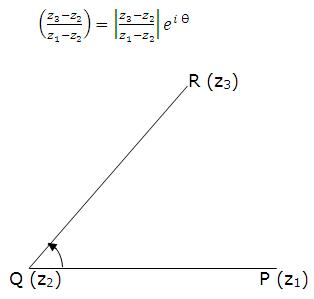(iii) If P(z1), Q(z2), R(z3) and S(z4) are three complex numbers and ⁄STQ= θ, then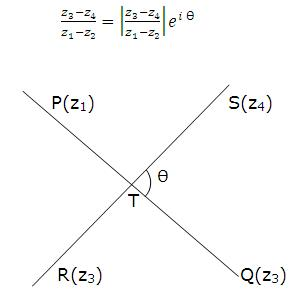2. amp (z) = θ is a ray emanating from the origin inclined at an angle θ to the x-axis.

3. |z-a|=|z-b| is the perpendicular bisectors of the line joining a to b.

4. The equation of a line joining z1 and z2 is given by z = z1 + t(z1-z2) where t is a real parameter.

5. z = z1 (1+it) where t is a real parameter is a line through the point z, and perpendicular to the line joining z, to the origin.

6. The equation of a line passing through z1 and z2 can be expressed in the determinant form asThis is also the condition for three complex numbers z, z1, z2 to b collinear.

7. The equation of a circle having centre z0 and radius p is:

|z-z0| = p or z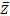- z0-0 - z +0z0 - p2 = 0 which is of the form

z+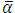z + α+ k = 0, k is real.

Centre is -α  and radius =√(α- k)  circle will be real if α- k ≥ 0.

8. The equation of the circle described on the line segment joining z1 and z2 as

diameter is arg (z-z2)/(z-z2 )= ±Π/2 or (z-z1) (-2)+(z-z2 )(1)=0

9. Condition for four given points z1, z2, z3 and z4 to be concyclic is the number

(z3 z1)/(z3-z2 ).(z4 z2)/(z4-z1 ) should be real.

Hence, the equation of a circle through 3 non-collinear points z1, z2 and z3 can be taken as

(z-z1 )(z3-z1 )/((z-z1 )(z3-z2)) is real

=>  (z-z2 )(z3-z1 )/((z-z1 )(z3-z2))=(-2 )(3-1)/((z-z1 )(z3-z2))

10. Arg((z-z1)/(z-z2)) = 0  represent

(i) a line segment if θ =Π

(ii) pair of ray if θ = 0

(iii) a part of circle, if 0<0<Π

11. Area of triangle formed by the points z1, z2 and z3 is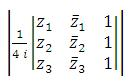12. Perpendicular distance of a point z0 from the linez + α+ r = 0  is |(z0 + α+ r )/α|α||

13. (i) Complex slope of a linez + α+ r = 0  w = - α/α

(ii) Complex slope of a line joining the points z1 and z2 is w=(z1-2)/(z1-2)

(iii) Complex slope of a line making Q angle with real axis w=l2iθ

14. Dot and Cross product

Let z1 = x1 + i y1 and z2 = x2 + i y2 be two complex numbers (vectors). The dot product (also called the scalar product) of z1 and z2 is defined by z1.z2 = |z1| |z2| cos θ

= x1x2 + y1y= = Re {1z2}

= 1/2{1z2 + z12}

Where θ is the angle between z1 and z2 which lies between 0 and Π .

If vectors z1, z2 are perpendicular then z1.z2 = 0 => z1/z2 + z2/z2 = 0

i.e. sum of complex slopes = 0

The cross product of z1 and z2 is defined by

z1 × z2 = |z1| |z2| sin θ|

= x1y2 - y1x2

= Im{1z2 }

= 1/zi (z2 - z12)

If vectors z1, z2 are parallel then z1 × z2 = 0 z1/z2 = z2/z2

i.e., complex slopes are equal.

Note:

w1 and w2 are complex slopes of two lines.

(i) If lines are parallel then w1 = w2

(ii) If lines are perpendicular then w1 + w2 = 0

15. If |z-z1| + |z-z2| = K> |z1-z2| then locus of z is an ellipse focii are z1 and z2.

16. If |z-z0| =  |z + α+ r/2K1| then locus of z is parabola whose focus is z0 and directrix is the linez + α+ r = 0 (providedz0+ α0 + r ≠ 0)

17. If |(z-z1)/(z-z2)| = k < |z1-z2|   then locus of z is a circle

18. If  then locus of z is a hyperbola, whose focii are z1 and z2.

To read more, Buy study materials of Complex Numbers comprising study notes, revision notes, video lectures, previous year solved questions etc. Also browse for more study materials on Mathematics here.
```### Course Features

• 731 Video Lectures
• Revision Notes
• Previous Year Papers
• Mind Map
• Study Planner
• NCERT Solutions
• Discussion Forum
• Test paper with Video Solution